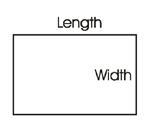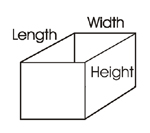# Pond Volume and Square Footage – Simple Calculations You Need To Know – Part 2 Rectangles

February 15, 2008CalculationsOK, here are moving on to rectangles.  FYI – all of these particular calculations will work for those of you with square ponds too.  As I said in part 1 of this series, you’re going to eventually need to know the total square footage of your pond as well as the volume.

The square footage will assist you in calculating how much of any construction material you’ll need to actually build the pond and the volume will allow you to calculate the needs of water that your pond holds.

Squares are pretty simple to calculate too.  Unlike circles, there are only three measurements you’ll need to take in order to get the information you need out of your calculations.  There is length, width and height.

• Length – This measurement is the longest extent of the pond, measured from end to end.
• Width – The measurement is the longest extent from side to side on the pond.
• Height – The measurement that is the longest extent from top to bottom of the pond.  You’d also refer to it as depth of the pond.When we calculated the square footage of the walls of a circular pond, there was only one wall.  So keep this in mind when calculating the square footage of a rectangular pond.  It has four walls and all need to be accounted for.  Let’s assume that the length of the pond is 24 feet, the width is 14 feet and the depth is 4.5 feet.

To calculate the total square footage of the floor you would multiply the length by the width like this,
24 (length) x 14 (width) = 336

So the floor has 336 square feet.

For the walls, you would multiply the length by the height for each wall and then add them all together.  So,
24 (length) x 4.5 (height) x 2 (walls) = 216
14 (width) x 4.5 (height) x 2 (walls) = 126

So for this rectangular pond, add the floor square footage to both wall square footage totals and you will end up with a total square footage for the entire pond.
336 (floor) + 216 (walls) + 126 (walls) = 678

Again, to calculate how much Pond Shield epoxy you would need to coat this pond, you divide the total square footage by 60 square feet.  Remember 60 square feet is the amount of coverage a quart and a half kits gives you at a minimal thickness of 10 mils.
678 / 60 = 11.3 (rounded up to 12 total quart and a half kits)

Now because we already know the length, width, and height, it will be very easy to calculate volume.  As a reminder, it is important to know the total volume of water your pond contains for purposes of water chemistry or how many fish you can safely house.  You know, that sort of thing.

To calculate volume of a rectangular shaped pond, you multiply the length by the width by the height of the pond like this,
24 (length) x 14 (width) x 4.5 (height) = 1512 cubic feet

Do you know how much all that water weighs?  12,700.8 pounds!  Yes, I make a big deal out of the total weight of the water.  I will tell you why.  If you are planning some sort of holding tank, something indoors, something on a pedestal of sort, weight becomes a serious issue and you need to know what that will be in the end.

Next time we will talk about ellipse shaped ponds and how to calculate square footage and volume for those.  I will give you a hint.  They are similar in nature to a circular pond, but do you know what the difference is?  I will let you know in part 3.